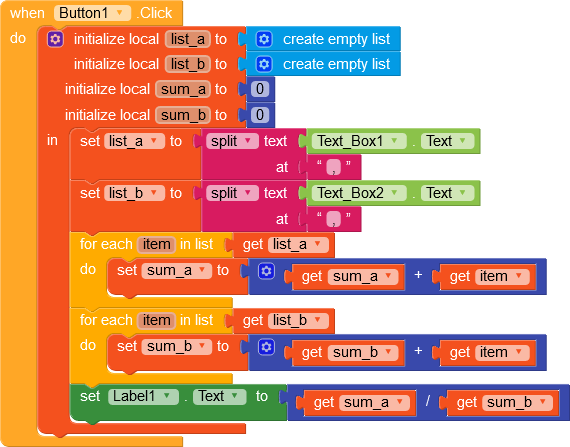# Calculating weighted average

Hello,
I’m new here and I would like to make an app which will calculate weighted average of given values but I would like user to put all values into 2 text boxes. Is it possible? For example textbox1.values are 10, 22, 35, 15 and textbox2.values are 2, 4, 1, 3 so my app will calculate them as =(102+224+351+153)/(10+22+35+15)

It is possible by creating lists from texboxes values using split text at `,` After that you can create a math procedure that calculates sum from first list, sum from second list and finally divide first sum by second sum

How can I create a list from textbox?

See an example below. This will work if textbox input is in form of `1,2,3,...` otherwise it won’t work2 Likes

This code sums the values in textbox1 and textbox2 but I need to multiply this values in an order and divided them to the sum of values in textbox1.

1 Like

Multiple wasn’t visible in your post

``````(10 *2+22* 4+35 *1+15* 3)/(10+22+35+15)`
``````

Try this

Sorry my fault. (10x2+22x4+35x1+15x3)/(10+22+35+15).

1 Like

My blocks are draggable, download them, drag them to block section and see if they work

I am so much grateful for your help. It worked. Thank You.

1 Like

This topic was automatically closed 30 days after the last reply. New replies are no longer allowed.